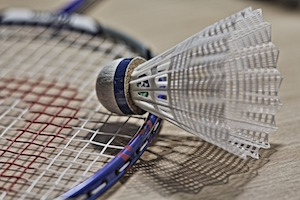Puzzle Editor Anirban DasGupta sets a problem on maximum likelihood estimation in a sports scenario.Between two badminton players $A$ and $B$, player $B$ has a probability $p_1 = p$ of returning a serve or a shot from $A$, and $A$ has a probability $p2 = cp$ of returning a serve or shot from $B$, where $c$ is a fixed positive constant. We make the usual independence assumption among shots. If $A$ fails to return a shot or a serve from $B$, the next serve is made by $B$, and vice versa. We also assume that the beginning serve in any play is always done successfully. Suppose $A$ begins the first play by serving to $B$. In 5 consecutive plays, the total number of shots successfully returned by the players before the first failure were: 8, 7, 0, 5, 9.

(a) Calculate the likelihood function.

(b) Find the maximum likelihood estimate of $p$.

(c) Take $p_1$ and $p_2$ to be general. $A$ serves to $B$ in the first play and B successfully returns the serve. But $A$ fails to return this volley from $B$. The serve now turns over to $B$, and $A$ is able to return the serve. But $B$ fails to return the volley from $A$. And so it goes on, for a total of $n$ plays — in other words, the number of shots successfully returned is always 1. What will happen to the MLEs of $p_1$ and $p_2$ in this scenario?

Student members of IMS are invited to submit solutions to bulletin@imstat.org (with subject “Student Puzzle Corner”). The names of student members who submit correct solutions, and the answer, will be published in the issue following the deadline.

The solution to puzzle 31 is here.